PHP Training

### Data Types and Operators

#### PHP Data Types

Variables can store data of different types, and different data types can do different things.

PHP supports the following data types:

• String
• Integer
• Float (floating point numbers - also called double)
• Boolean
• Array
• Object
• NULL
• Resource

#### PHP String

A string is a sequence of characters, like "Hello world!".

A string can be any text inside quotes. You can use single or double quotes:

### Example

<?php
\$x = "Hello world!";
\$y = 'Hello world!';

echo \$x;
echo "<br>";
echo \$y;
?>
//Output:
Hello world!
Hello world!

#### PHP Integer

An integer data type is a non-decimal number between -2,147,483,648 and 2,147,483,647.

Rules for integers:

• An integer must have at least one digit
• An integer must not have a decimal point
• An integer can be either positive or negative
• Integers can be specified in three formats: decimal (10-based), hexadecimal (16-based - prefixed with 0x) or octal (8-based - prefixed with 0)

In the following example \$x is an integer. The PHP var_dump() function returns the data type and value:

### Example

<?php
\$x = 5985;
var_dump(\$x);
?>
//Output:
int(5985)

#### PHP Float

A float (floating point number) is a number with a decimal point or a number in exponential form.

In the following example \$x is a float. The PHP var_dump() function returns the data type and value:

### Example

<?php
\$x = 10.365;
var_dump(\$x);
?>
//Output:
float(10.365)

#### PHP Boolean

A Boolean represents two possible states: TRUE or FALSE.

\$x = true;
\$y = false;

Booleans are often used in conditional testing. You will learn more about conditional testing in a later chapter of this tutorial.

#### PHP Array

An array stores multiple values in one single variable.

In the following example \$cars is an array. The PHP var_dump() function returns the data type and value:

### Example

<?php
\$cars = array("Volvo","BMW","Toyota");
var_dump(\$cars);
?>
//Output:
array(3) { => string(5) "Volvo" => string(3) "BMW" => string(6) "Toyota" }

#### PHP Object

An object is a data type which stores data and information on how to process that data.

In PHP, an object must be explicitly declared.

First we must declare a class of object. For this, we use the class keyword. A class is a structure that can contain properties and methods:

### Example

<?php
class Car {
function Car() {
\$this->model = "VW";
}
}

// create an object
\$herbie = new Car();

// show object properties
echo \$herbie->model;
?>
//Output:
VW

#### PHP NULL Value

Null is a special data type which can have only one value: NULL.

A variable of data type NULL is a variable that has no value assigned to it.

Tip: If a variable is created without a value, it is automatically assigned a value of NULL.

Variables can also be emptied by setting the value to NULL:

### Example

<?php
\$x = "Hello world!";
\$x = null;
var_dump(\$x);
?>
//Output:
NULL

#### PHP Resource

The special resource type is not an actual data type. It is the storing of a reference to functions and resources external to PHP.

A common example of using the resource data type is a database call.

#### Strings

A string is a sequence of characters, like "Hello world!".

The PHP strlen() function returns the length of a string.

The example below returns the length of the string "Hello world!":

### Example

<?php
echo strlen("Hello world!"); // Output: 12
?>

The output of the code above will be: 12.

#### Count The Number of Words in a String

The PHP str_word_count() function counts the number of words in a string:

### Example

<?php
echo str_word_count("Hello world!"); // Output: 2
?>

The output of the code above will be: 2.

#### Reverse a String

The PHP strrev() function reverses a string:

### Example

<?php
echo strrev("Hello world!"); // Output: !dlrow olleH
?>

The output of the code above will be: !dlrow olleH.

#### Search For a Specific Text Within a String

The PHP strpos() function searches for a specific text within a string.

If a match is found, the function returns the character position of the first match. If no match is found, it will return FALSE.

The example below searches for the text "world" in the string "Hello world!":

### Example

<?php
echo strpos("Hello world!", "world"); // Output: 6
?>

The output of the code above will be: 6.

Tip: The first character position in a string is 0 (not 1).

#### Replace Text Within a String

The PHP str_replace() function replaces some characters with some other characters in a string.

The example below replaces the text "world" with "Dolly":

### Example

<?php
echo str_replace("world", "Dolly", "Hello world!"); // Output: Hello Dolly!
?>

The output of the code above will be: Hello Dolly!

#### PHP Constants

Constants are like variables except that once they are defined they cannot be changed or undefined.

A constant is an identifier (name) for a simple value. The value cannot be changed during the script.

A valid constant name starts with a letter or underscore (no \$ sign before the constant name).

Note: Unlike variables, constants are automatically global across the entire script.

#### Create a PHP Constant

To create a constant, use the define() function.

### Syntax

define(name, value, case-insensitive)

Parameters:

• name: Specifies the name of the constant
• value: Specifies the value of the constant
• case-insensitive: Specifies whether the constant name should be case-insensitive. Default is false

The example below creates a constant with a case-sensitive name:

### Example

<?php
define("GREETING", "Welcome to W3Schools.com!");
echo GREETING;
?>
//Output:
Welcome to W3Schools.com!

The example below creates a constant with a case-insensitive name:

### Example

<?php
define("GREETING", "Welcome to W3Schools.com!", true);
echo greeting;
?>
//Output:
Welcome to W3Schools.com!

#### Constants are Global

Constants are automatically global and can be used across the entire script.

The example below uses a constant inside a function, even if it is defined outside the function:

### Example

<?php
define("GREETING", "Welcome to W3Schools.com!");

function myTest() {
echo GREETING;
}

myTest();
?>
//Output:
Welcome to W3Schools.com!

#### PHP Operators

Operators are used to perform operations on variables and values.

PHP divides the operators in the following groups:

• Arithmetic operators
• Assignment operators
• Comparison operators
• Increment/Decrement operators
• Logical operators
• String operators
• Array operators

#### PHP Arithmetic Operators

The PHP arithmetic operators are used with numeric values to perform common arithmetical operations, such as addition, subtraction, multiplication etc.

Operator Name Example Result
+ Addition \$x + \$y Sum of \$x and \$y
- Subtraction \$x - \$y Difference of \$x and \$y
* Multiplication \$x * \$y Product of \$x and \$y
/ Division \$x / \$y Quotient of \$x and \$y
% Modulus \$x % \$y Remainder of \$x divided by \$y
** Exponentiation \$x ** \$y Result of raising \$x to the \$y'th power (Introduced in PHP 5.6)

#### PHP Assignment Operators

The PHP assignment operators are used with numeric values to write a value to a variable.

The basic assignment operator in PHP is "=". It means that the left operand gets set to the value of the assignment expression on the right.

Assignment Same as... Description
x = y x = y The left operand gets set to the value of the expression on the right
x += y x = x + y Addition
x -= y x = x - y Subtraction
x *= y x = x * y Multiplication
x /= y x = x / y Division
x %= y x = x % y Modulus

#### PHP Comparison Operators

The PHP comparison operators are used to compare two values (number or string):

Operator Name Example Result
== Equal \$x == \$y Returns true if \$x is equal to \$y
=== Identical \$x === \$y Returns true if \$x is equal to \$y, and they are of the same type
!= Not equal \$x != \$y Returns true if \$x is not equal to \$y
<> Not equal \$x <> \$y Returns true if \$x is not equal to \$y
!== Not identical \$x !== \$y Returns true if \$x is not equal to \$y, or they are not of the same type
> Greater than \$x > \$y Returns true if \$x is greater than \$y
< Less than \$x < \$y Returns true if \$x is less than \$y
>= Greater than or equal to \$x >= \$y Returns true if \$x is greater than or equal to \$y
<= Less than or equal to \$x <= \$y Returns true if \$x is less than or equal to \$y

#### PHP Increment / Decrement Operators

The PHP increment operators are used to increment a variable's value.

The PHP decrement operators are used to decrement a variable's value.

Operator Name Description
++\$x Pre-increment Increments \$x by one, then returns \$x
\$x++ Post-increment Returns \$x, then increments \$x by one
--\$x Pre-decrement Decrements \$x by one, then returns \$x
\$x-- Post-decrement Returns \$x, then decrements \$x by one

#### PHP Logical Operators

The PHP logical operators are used to combine conditional statements.

Operator Name Example Result
and And \$x and \$y True if both \$x and \$y are true
or Or \$x or \$y True if either \$x or \$y is true
xor Xor \$x xor \$y True if either \$x or \$y is true, but not both
&& And \$x && \$y True if both \$x and \$y are true
|| Or \$x || \$y True if either \$x or \$y is true
! Not !\$x True if \$x is not true

#### PHP String Operators

PHP has two operators that are specially designed for strings.

Operator Name Example Result
. Concatenation \$txt1 . \$txt2 Concatenation of \$txt1 and \$txt2
.= Concatenation assignment \$txt1 .= \$txt2 Appends \$txt2 to \$txt1

#### PHP Array Operators

The PHP array operators are used to compare arrays.

Operator Name Example Result
+ Union \$x + \$y Union of \$x and \$y
== Equality \$x == \$y Returns true if \$x and \$y have the same key/value pairs
=== Identity \$x === \$y Returns true if \$x and \$y have the same key/value pairs in the same order and of the same types
!= Inequality \$x != \$y Returns true if \$x is not equal to \$y
<> Inequality \$x <> \$y Returns true if \$x is not equal to \$y
!== Non-identity \$x !== \$y Returns true if \$x is not identical to \$y

#### Precedence of PHP Operators

Operator precedence determines the grouping of terms in an expression. This affects how an expression is evaluated. Certain operators have higher precedence than others; for example, the multiplication operator has higher precedence than the addition operator −

For example x = 7 + 3 * 2; Here x is assigned 13, not 20 because operator * has higher precedence than + so it first get multiplied with 3*2 and then adds into 7.

Here operators with the highest precedence appear at the top of the table, those with the lowest appear at the bottom. Within an expression, higher precedence operators will be evaluated first.

Category Operator Associativity
Unary ! ++ -- Right to left
Multiplicative * / % Left to right
Additive + - Left to right
Relational < <= > >= Left to right
Equality == != Left to right
Logical AND && Left to right
Logical OR || Left to right
Conditional ?: Right to left
Assignment = += -= *= /= %= Right to left

### PHP Workflow

( 0 Review )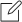### Course Code :

02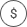### Price :

5000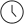### Duration :

30 days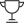### Lectures :

10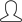### Students :

10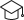### Certificate of Completion### Manish Kumar Shrivastava

Web Developer

My name is Ruth. I grew up and studied in…